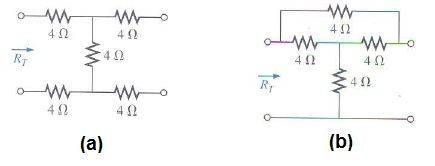# Resistancein a circuit ,,

• Lord Dark

#### Lord Dark

Resistance in a circuit ,,

## Homework Statement

Hi guys ,, I have the following picture## The Attempt at a Solution

OK ,, I know it's easy but my answer's is not like my teacher's answer :

for (a) I get 6.12 ohm and for (b) I get 2.4 ohm

but my teacher's answer is :

a. Rt=4+4+4=12 ohm
b. Rt= (8//4)+4= 6.667 ohm

what I don't get for :

a. how can he just add them together ,, it's not like they are in series and 2 of them are in parallel .. and I don't know why adding only 3 resistors only ?? there are 5 ~.~ ,,

b.how come 8//4 and there is a resistor between 4 & 4 ,, so don't we have to take a parallel (4//4) then add with 4 then parallel them with the upper resistor ??

so can someone simplify circuits please -_- ,, (I usually use current direction to know whether they are in parallel or series)

#### Attachments

Last edited:
Think about which wires in the circuit that would actually carry a current if you were to measure R_T by applying a voltage over the two left terminals. It may also help to redraw the diagram so you have the top-left terminal on the left and the bottom left terminal on the right.

aha ,, now I get it ,, so the arrow (Rt) in the diagram means where the voltage terminals are ,, I get it for (a) and (b) too ,, thanks very much filiplarsen ,, but question , if both circuits where closed my answers were right ??

If you by closed mean that the two terminals to the right are short-circuited, then I get
a) 4+(8//4)+4 = 10 2/3, and
b) 4//(4+(4//4)) = 4//6 = 2 2/5
So we have same result for b) but not a).

Lol,, got it :)

thanks very much m8